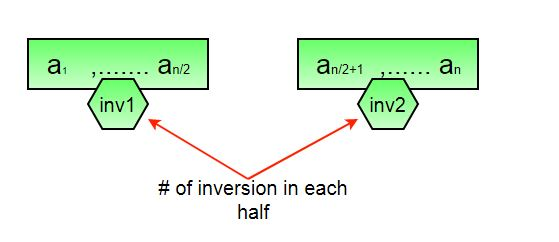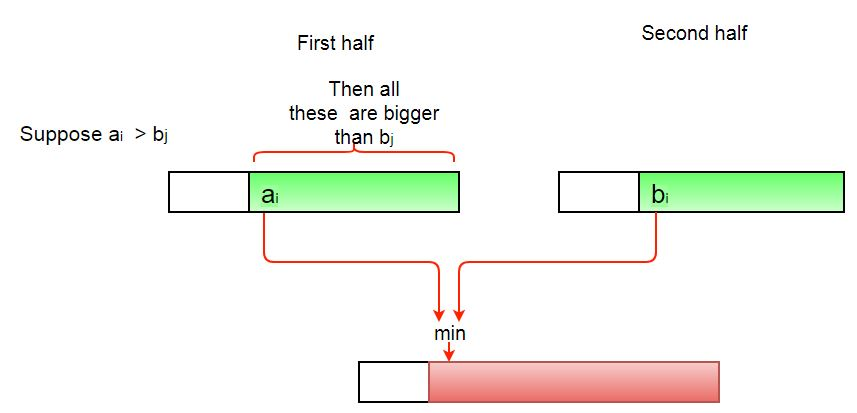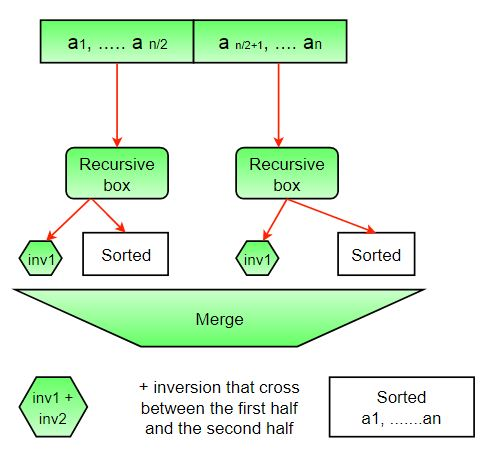GFG App
Open AppBrowser
Continue

# C++ Program For Counting Inversions In An Array – Set 1 (Using Merge Sort)

Inversion Count for an array indicates – how far (or close) the array is from being sorted. If the array is already sorted, then the inversion count is 0, but if the array is sorted in the reverse order, the inversion count is the maximum.
Formally speaking, two elements a[i] and a[j] form an inversion if a[i] > a[j] and i < j
Example:

```Input: arr[] = {8, 4, 2, 1}
Output: 6
Explanation: Given array has six inversions:
(8, 4), (4, 2), (8, 2), (8, 1), (4, 1), (2, 1).

Input: arr[] = {3, 1, 2}
Output: 2
Explanation: Given array has two inversions:
(3, 1), (3, 2) ```

METHOD 1 (Simple):

Approach: Traverse through the array, and for every index, find the number of smaller elements on its right side of the array. This can be done using a nested loop. Sum up the counts for all index in the array and print the sum.

Algorithm:

1. Traverse through the array from start to end
2. For every element, find the count of elements smaller than the current number up to that index using another loop.
3. Sum up the count of inversion for every index.
4. Print the count of inversions.

Implementation:

## C++

 `// C++ program to Count Inversions ` `// in an array ` `#include ` `using` `namespace` `std; ` ` `  `int` `getInvCount(``int` `arr[], ``int` `n) ` `{ ` `    ``int` `inv_count = 0; ` `    ``for` `(``int` `i = 0; i < n - 1; i++) ` `        ``for` `(``int` `j = i + 1; j < n; j++) ` `            ``if` `(arr[i] > arr[j]) ` `                ``inv_count++; ` ` `  `    ``return` `inv_count; ` `} ` ` `  `// Driver Code ` `int` `main() ` `{ ` `    ``int` `arr[] = {1, 20, 6, 4, 5}; ` `    ``int` `n = ``sizeof``(arr) / ``sizeof``(arr); ` `    ``cout << ``" Number of inversions are "` `<<  ` `              ``getInvCount(arr, n); ` `    ``return` `0; ` `} ` `// This code is contributed by Akanksha Rai `

Output:

` Number of inversions are 5`

Complexity Analysis:

• Time Complexity: O(n^2), Two nested loops are needed to traverse the array from start to end, so the Time complexity is O(n^2)
• Space Complexity:O(1), No extra space is required.

METHOD 2(Enhance Merge Sort):

Approach:
Suppose the number of inversions in the left half and right half of the array (let be inv1 and inv2); what kinds of inversions are not accounted for in Inv1 + Inv2? The answer is – the inversions that need to be counted during the merge step. Therefore, to get the total number of inversions that needs to be added are the number of inversions in the left subarray, right subarray, and merge().How to get the number of inversions in merge()?
In merge process, let i is used for indexing left sub-array and j for right sub-array. At any step in merge(), if a[i] is greater than a[j], then there are (mid – i) inversions. because left and right subarrays are sorted, so all the remaining elements in left-subarray (a[i+1], a[i+2] … a[mid]) will be greater than a[j]The complete picture:Algorithm:

1. The idea is similar to merge sort, divide the array into two equal or almost equal halves in each step until the base case is reached.
2. Create a function merge that counts the number of inversions when two halves of the array are merged, create two indices i and j, i is the index for the first half, and j is an index of the second half. if a[i] is greater than a[j], then there are (mid – i) inversions. because left and right subarrays are sorted, so all the remaining elements in left-subarray (a[i+1], a[i+2] … a[mid]) will be greater than a[j].
3. Create a recursive function to divide the array into halves and find the answer by summing the number of inversions is the first half, the number of inversion in the second half and the number of inversions by merging the two.
4. The base case of recursion is when there is only one element in the given half.

Implementation:

## C++

 `// C++ program to Count Inversions in  ` `// an array using Merge Sort ` `#include ` `using` `namespace` `std; ` ` `  `int` `_mergeSort(``int` `arr[], ``int` `temp[],  ` `               ``int` `left, ``int` `right); ` `int` `merge(``int` `arr[], ``int` `temp[],  ` `          ``int` `left, ``int` `mid, ``int` `right); ` ` `  `/* This function sorts the input  ` `   ``array and returns the number  ` `   ``of inversions in the array */` `int` `mergeSort(``int` `arr[], ``int` `array_size) ` `{ ` `    ``int` `temp[array_size]; ` `    ``return` `_mergeSort(arr, temp, 0,  ` `                      ``array_size - 1); ` `} ` ` `  `/* An auxiliary recursive function that  ` `   ``sorts the input array and returns the  ` `   ``number of inversions in the array. */` `int` `_mergeSort(``int` `arr[], ``int` `temp[],  ` `               ``int` `left, ``int` `right) ` `{ ` `    ``int` `mid, inv_count = 0; ` `    ``if` `(right > left)  ` `    ``{ ` `        ``/* Divide the array into two parts and  ` `           ``call _mergeSortAndCountInv() for  ` `           ``each of the parts */` `        ``mid = (right + left) / 2; ` ` `  `        ``/* Inversion count will be sum of inversions  ` `           ``in left-part, right-part and number of  ` `           ``inversions in merging */` `        ``inv_count += _mergeSort(arr, temp,  ` `                                ``left, mid); ` `        ``inv_count += _mergeSort(arr, temp,  ` `                                ``mid + 1, right); ` ` `  `        ``// Merge the two parts ` `        ``inv_count += merge(arr, temp,  ` `                           ``left, mid + 1, right); ` `    ``} ` `    ``return` `inv_count; ` `} ` ` `  `/* This funt merges two sorted arrays and  ` `   ``returns inversion count in the arrays.*/` `int` `merge(``int` `arr[], ``int` `temp[],  ` `          ``int` `left, ``int` `mid, ``int` `right) ` `{ ` `    ``int` `i, j, k; ` `    ``int` `inv_count = 0; ` ` `  `    ``// i is index for left subarray ` `    ``i = left; ` ` `  `    ``// j is index for right subarray  ` `    ``j = mid;  ` ` `  `    ``// k is index for resultant merged  ` `    ``// subarray ` `    ``k = left;  ` ` `  `    ``while` `((i <= mid - 1) &&  ` `           ``(j <= right))  ` `    ``{ ` `        ``if` `(arr[i] <= arr[j])  ` `        ``{ ` `            ``temp[k++] = arr[i++]; ` `        ``} ` `        ``else`  `        ``{ ` `            ``temp[k++] = arr[j++]; ` ` `  `            ``/* This is tricky -- see above  ` `               ``explanation/diagram for merge()*/` `            ``inv_count = inv_count + (mid - i); ` `        ``} ` `    ``} ` ` `  `    ``/* Copy the remaining elements of left  ` `       ``subarray (if there are any) to temp*/` `    ``while` `(i <= mid - 1) ` `        ``temp[k++] = arr[i++]; ` ` `  `    ``/* Copy the remaining elements of right  ` `       ``subarray (if there are any) to temp*/` `    ``while` `(j <= right) ` `        ``temp[k++] = arr[j++]; ` ` `  `    ``/* Copy back the merged elements to  ` `       ``original array*/` `    ``for` `(i = left; i <= right; i++) ` `        ``arr[i] = temp[i]; ` ` `  `    ``return` `inv_count; ` `} ` ` `  `// Driver code ` `int` `main() ` `{ ` `    ``int` `arr[] = {1, 20, 6, 4, 5}; ` `    ``int` `n = ``sizeof``(arr) / ``sizeof``(arr); ` `    ``int` `ans = mergeSort(arr, n); ` `    ``cout << ``" Number of inversions are "` `<< ans; ` `    ``return` `0; ` `} ` ` `  `// This is code is contributed by rathbhupendra `

Output:

`Number of inversions are 5`

Complexity Analysis:

• Time Complexity: O(n log n), The algorithm used is divide and conquer, So in each level, one full array traversal is needed, and there are log n levels, so the time complexity is O(n log n).
• Space Complexity: O(n), Temporary array.

Note that the above code modifies (or sorts) the input array. If we want to count only inversions, we need to create a copy of the original array and call mergeSort() on the copy to preserve the original array’s order.

My Personal Notes arrow_drop_up